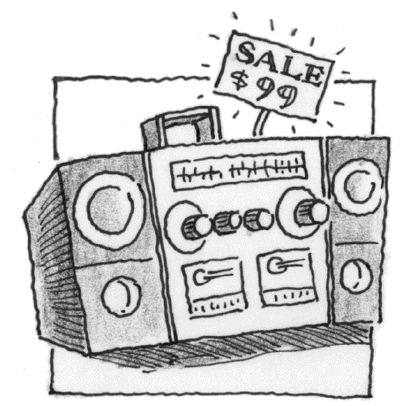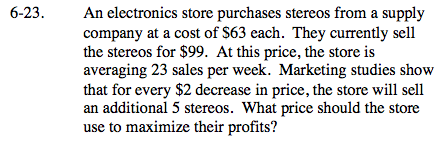### Home > CALC > Chapter 6 > Lesson 6.1.2 > Problem6-23

6-23.An electronics store purchases stereos from a supply company at a cost of $63 each. They currently sell the stereos for$99. At this price, the store is averaging 23 sales per week. Marketing studies show that for every $2 decrease in price, the store will sell an additional 5 stereos. What price should the store use to maximize their profits? Homework Help ✎Revenue = (profit)(number sold) Calculate the current revenue: Profit:$99 - $63 Number sold: 23 Adjust the current revenue equation: Let x = the number of times the price is decreased by$2.

R(x) = (36 − 2x)(23 + 5x)

Optimize (that is, use derivatives to find the optimal value of x). But note, the question is asking about price, not x.